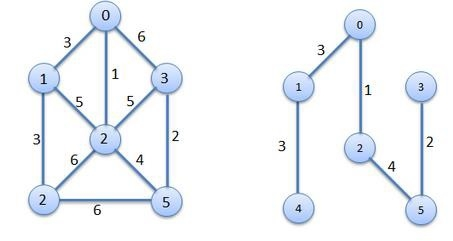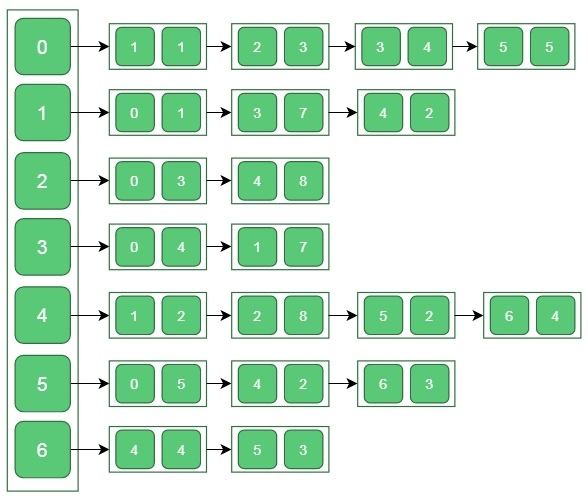# Prim’s (Minimum Spanning Tree) MST Algorithm

There is a connected graph G(V,E) and the weight or cost for every edge is given. Prim’s Algorithm will find the minimum spanning tree from the graph G.

It is growing tree approach. This algorithm needs a seed value to start the tree. The seed vertex is grown to form the whole tree.The problem will be solved using two sets. One set holds the nodes that are already selected, and another set holds the item that are not considered yet. From the seed vertex, it takes adjacent vertices, based on minimum edge cost, thus it grows the tree by taking nodes one by one.

• Time complexity of this problem is O(V2). Here V is the number of vertices.

Input − The adjacency list −Output −

(0)---(1|1) (0)---(2|3) (0)---(3|4)
(1)---(0|1) (1)---(4|2)
(2)---(0|3)
(3)---(0|4)
(4)---(1|2) (4)---(5|2)
(5)---(4|2) (5)---(6|3)
(6)---(5|3)

## Algorithm

### prims(g: Graph, t: tree, start)

Input − The graph g, A blank tree and the seed vertex named ‘start’ Output: The Tree after adding edges.

Begin
define two sets as usedVert, unusedVert
usedVert := start and unusedVert := φ
for all vertices except start do
usedVert[i] := φ
unusedVert[i] := i //add all vertices in unused list
done
while number of vertices in usedVert ≠ V do //V is number of total nodes
min := ∞
for all vertices of usedVert array do
for all vertices of the graph do
if min > cost[i,j] AND i ≠ j then
min := cost[i,j]
ed := edge between i and j, and cost of ed := min
done
done
unusedVert[destination of ed] := φ
add edge ed into the tree t
add source of ed into usedVert
done
End

## Example(C++)

#include<iostream>
#define V 7
#define INF 999
using namespace std;
//Cost matrix of the graph
int costMat[V][V] = {
{0, 1, 3, 4, INF, 5, INF},
{1, 0, INF, 7, 2, INF, INF},
{3, INF, 0, INF, 8, INF, INF},
{4, 7, INF, 0, INF, INF, INF},
{INF, 2, 8, INF, 0, 2, 4},
{5, INF, INF, INF, 2, 0, 3},
{INF, INF, INF, INF, 4, 3, 0}
};
typedef struct{
int u, v, cost;
}edge;
class Tree{
int n;
edge edges[V-1]; //as a tree has vertex-1 edges
public:
Tree(){
n = 0;
}
edges[n] = e; //add edge e into the tree
n++;
}
void printEdges(){ //print edge, cost and total cost
int tCost = 0;
for(int i = 0; i<n; i++){
cout << "Edge: " << char(edges[i].u+'A') < "--" << char(edges[i].v+'A');
cout << " And Cost: " << edges[i].cost << endl;
tCost += edges[i].cost;
}
cout << "Total Cost: " << tCost << endl;
}
friend void prims(Tree &tre, int start);
};
void prims(Tree &tr, int start){
int usedVert[V], unusedVert[V];
int i, j, min, p;
edge ed;
//initialize
usedVert = start; p = 1;
unusedVert = -1;//-1 indicates the place is empty
for(i = 1; i<V; i++){
usedVert[i] = -1;//all places except first is empty
unusedVert[i] = i;//fill with vertices
}
tr.n = 0;
//get edges and add to tree
while(p != V){ //p is number of vertices in usedVert array
min = INF;
for(i = 0; i<p; i++){
for(j = 0; j<V; j++){
if(unusedVert[j] != -1){
if(min > costMat[i][j] && costMat[i][j] != 0){
//find the edge with minimum cost
//such that u is considered and v is not considered yet
min = costMat[i][j];
ed.u = i; ed.v = j; ed.cost = min;
}
}
}
}
unusedVert[ed.v] = -1;//delete v from unusedVertex
usedVert[p] = ed.u; p++;//add u to usedVertex
}
}
main(){
Tree tr;
prims(tr, 0); //starting node 0
tr.printEdges();
}

## Output

(0)---(1|1) (0)---(2|3) (0)---(3|4)
(1)---(0|1) (1)---(4|2)
(2)---(0|3)
(3)---(0|4)
(4)---(1|2) (4)---(5|2)
(5)---(4|2) (5)---(6|3)
(6)---(5|3)